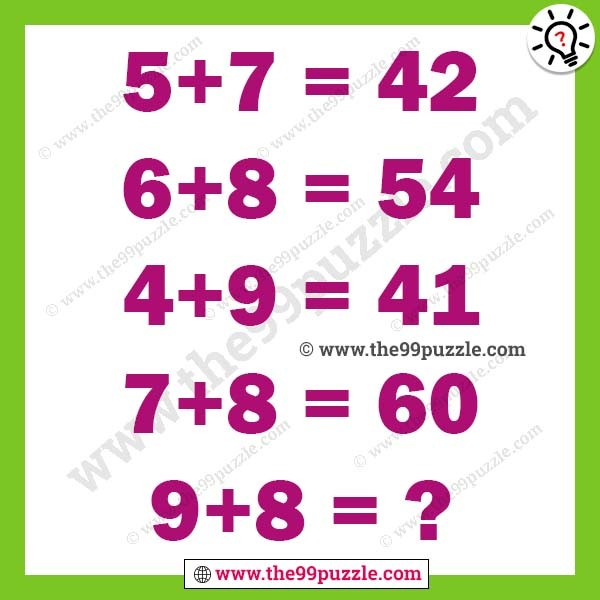# Logical math equation with answer for teens – Puzz123

The logical math equation will challenge you to find the value. In this intelligence test, your challenge is to find the logic used in the given logical math equation. If you are a genius minded you will solve this puzzle very quickly.

5+7=42

6+8=54

4+9=41

7+8=60

9+8=?5+7=5×7+7=42

6+8=6×8+6=54

4+9=4×9+5=41

7+8=7×8+4=60

9+8=9×8+3=75